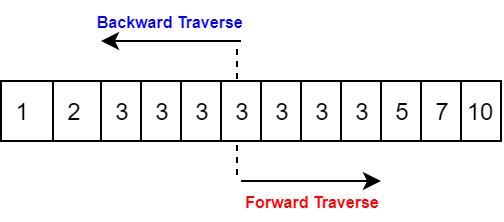# Search For Range

## Problem

Given a sorted array of integers, find the starting and ending position of a given target value. Your algorithm’s runtime complexity must be in the order of O(log n) . If the target is not found in the array, return [-1, -1] .

### Example

Input : [0,1,3,3,3,3,3,3,3,3,10] , value = 3
Output: [2,9]

Input : [5,7,7,8,8,10] , value = 8
Output: [3,4]

Input : [] , value = 7
Output: [-1,-1]

Input : [1,3,5,6] , value = 5
Output: [2,2]


## Analysis

The problem forces us to think in BinarySearch algorithm’s term, since the time complexity myst be in order of O(log n) . We can design an algorithm using Binary search to get to the first occurance of element, if found we can traverse teh array backward and forward to find the start and end of the range which will add another O(m+k)Happy Coding!

Tags:

Updated: